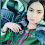In this article, I am going to explain how to merge two excel files based their header values. If excel file is having large number of rows and columns with disordered column types  then it will be difficult to merge them. If you want to do any modification by logic in merging, you can do using this program easily. To implement this program you need to download Apache POI library and have it in your build path (copy POI jars to lib folder).
There is no direct method to merge excel files using java. You need to follow the below flow.

### Flow Of The Program

1. Get Excel files
2. Get Work Books of those excel files
3. Get sheets to be merged in those excel files
4. Create Map based on headers of given excel sheets
6. Read every cell and add it to the row based on Map
7. Write merged workbook to output file

### Java Code

```import java.io.File;
import java.io.FileInputStream;
import java.io.FileOutputStream;
import java.util.HashMap;
import java.util.Map;
import java.util.Set;
import org.apache.poi.hssf.usermodel.HSSFCell;
import org.apache.poi.xssf.usermodel.XSSFCell;
import org.apache.poi.xssf.usermodel.XSSFCellStyle;
import org.apache.poi.xssf.usermodel.XSSFRow;
import org.apache.poi.xssf.usermodel.XSSFSheet;
import org.apache.poi.xssf.usermodel.XSSFWorkbook;

public static void main(String[] args) {
try {
// get input excel files
FileInputStream excellFile1 = new FileInputStream(new File(
"C:\\inputExcel1.xlsx"));
FileInputStream excellFile2 = new FileInputStream(new File(
"C:\\inputExcel2.xlsx"));

// Create Workbook instance holding reference to .xlsx file
XSSFWorkbook workbook1 = new XSSFWorkbook(excellFile1);
XSSFWorkbook workbook2 = new XSSFWorkbook(excellFile2);

// Get first/desired sheet from the workbook
XSSFSheet mainSheet = workbook1.getSheetAt(0);
XSSFSheet sheet2 = workbook2.getSheetAt(0);

excellFile1.close();
excellFile2.close();

// save merged file
File mergedFile = new File("C:\\merged.xlsx");
if (!mergedFile.exists()) {
mergedFile.createNewFile();
}
FileOutputStream out = new FileOutputStream(mergedFile);
workbook1.write(out);
out.close();
System.out.println("Files were merged succussfully");
} catch (Exception e) {
e.printStackTrace();
}

}

public static void addSheet(XSSFSheet mainSheet, XSSFSheet sheet, HashMap<Integer, Integer> map) {

//get column number
Set<Integer> colNumbs = map.keySet();
// map for cell styles
Map<Integer, XSSFCellStyle> styleMap = new HashMap<Integer, XSSFCellStyle>();

// This parameter is for appending sheet rows to mergedSheet in the end
int len = mainSheet.getLastRowNum();
for (int j = sheet.getFirstRowNum() + 1 ; j <= sheet.getLastRowNum(); j++) {

XSSFRow row = sheet.getRow(j);

// Create row in main sheet
XSSFRow mrow = mainSheet.createRow(len + j);

for (Integer k : colNumbs) {
XSSFCell cell = row.getCell(k.intValue());

// Create column in main sheet
XSSFCell mcell = mrow.createCell(map.get(k).intValue());

if (cell.getSheet().getWorkbook() == mcell.getSheet()
.getWorkbook()) {
mcell.setCellStyle(cell.getCellStyle());
} else {
int stHashCode = cell.getCellStyle().hashCode();
XSSFCellStyle newCellStyle = styleMap.get(stHashCode);
if (newCellStyle == null) {
newCellStyle = mcell.getSheet().getWorkbook()
.createCellStyle();
newCellStyle.cloneStyleFrom(cell.getCellStyle());
styleMap.put(stHashCode, newCellStyle);
}
mcell.setCellStyle(newCellStyle);
}

// set value based on cell type
switch (cell.getCellType()) {
case HSSFCell.CELL_TYPE_FORMULA:
mcell.setCellFormula(cell.getCellFormula());
break;
case HSSFCell.CELL_TYPE_NUMERIC:
mcell.setCellValue(cell.getNumericCellValue());
break;
case HSSFCell.CELL_TYPE_STRING:
mcell.setCellValue(cell.getStringCellValue());
break;
case HSSFCell.CELL_TYPE_BLANK:
mcell.setCellType(HSSFCell.CELL_TYPE_BLANK);
break;
case HSSFCell.CELL_TYPE_BOOLEAN:
mcell.setCellValue(cell.getBooleanCellValue());
break;
case HSSFCell.CELL_TYPE_ERROR:
mcell.setCellErrorValue(cell.getErrorCellValue());
break;
default:
mcell.setCellValue(cell.getStringCellValue());
break;
}
}
}
}

// results MAP of <secondary sheet, main sheet>
public static HashMap<Integer, Integer> mapHeaders(XSSFSheet sheet1,
XSSFSheet sheet2) {
HashMap<Integer, Integer> map = new HashMap<Integer, Integer>();
XSSFRow row1 = sheet1.getRow(0);
XSSFRow row2 = sheet2.getRow(0);
for (int i = row1.getFirstCellNum(); i < row1.getLastCellNum(); i++) {
for (int j = row2.getFirstCellNum(); j < row2.getLastCellNum(); j++) {
if (row1.getCell(i).getStringCellValue()
.equals(row2.getCell(j).getStringCellValue())) {
map.put(new Integer(i), new Integer(j));
}
}
}
return map;
}
}
```

1.Thanks for sharing this informative post on your blog. i think every person not able to write java code for merge excel files. i am using Free Software (Synkronizer Excel Tool) for merge multiple files without writing any java code or script.

2.This comment has been removed by the author.

3.animal feed testing - Alfa Chemistry is an analytical testing company that provides one-stop testing, analysis and technical support services in the areas of pharmaceutical analysis, environmental analysis, consumer product analysis, food analysis, and material analysis.

## BlogrollSrinivas Dasari find me on facebook follow me on twitter